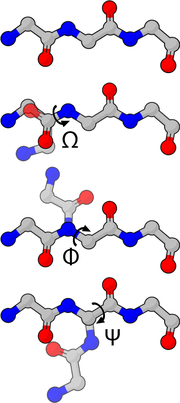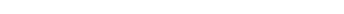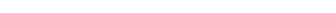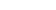xMolecular modellingEncyclopediaMolecular modelling encompasses all theoretical methods and computational techniques used to model or mimic the behaviour of molecule
Molecule
A molecule is an electrically neutral group of at least two atoms held together by covalent chemical bonds. Molecules are distinguished from ions by their electrical charge...

s. The techniques are used in the fields of computational chemistry
Computational chemistry
Computational chemistry is a branch of chemistry that uses principles of computer science to assist in solving chemical problems. It uses the results of theoretical chemistry, incorporated into efficient computer programs, to calculate the structures and properties of molecules and solids...

, computational biology
Computational biology
Computational biology involves the development and application of data-analytical and theoretical methods, mathematical modeling and computational simulation techniques to the study of biological, behavioral, and social systems...

and materials science
Materials science
Materials science is an interdisciplinary field applying the properties of matter to various areas of science and engineering. This scientific field investigates the relationship between the structure of materials at atomic or molecular scales and their macroscopic properties. It incorporates...

for studying molecular systems ranging from small chemical systems to large biological molecules and material assemblies. The simplest calculations can be performed by hand, but inevitably computers are required to perform molecular modelling of any reasonably sized system. The common feature of molecular modelling techniques is the atomistic level description of the molecular systems; the lowest level of information is individual atoms (or a small group of atoms). This is in contrast to quantum chemistry
Quantum chemistry
Quantum chemistry is a branch of chemistry whose primary focus is the application of quantum mechanics in physical models and experiments of chemical systems...

(also known as electronic structure calculations) where electrons are considered explicitly. The benefit of molecular modelling is that it reduces the complexity of the system, allowing many more particles (atoms) to be considered during simulations.

## Molecular mechanics

Molecular mechanics
Molecular mechanics
Molecular mechanics uses Newtonian mechanics to model molecular systems. The potential energy of all systems in molecular mechanics is calculated using force fields...

is one aspect of molecular modelling, as it refers to the use of classical mechanics
Classical mechanics
In physics, classical mechanics is one of the two major sub-fields of mechanics, which is concerned with the set of physical laws describing the motion of bodies under the action of a system of forces...

/Newtonian mechanics to describe the physical basis behind the models. Molecular models typically describe atoms (nucleus and electrons collectively) as point charges with an associated mass. The interactions between neighbouring atoms are described by spring-like interactions (representing chemical bonds) and van der Waals forces. The Lennard-Jones potential
Lennard-Jones potential
The Lennard-Jones potential is a mathematically simple model that approximates the interaction between a pair of neutral atoms or molecules. A form of the potential was first proposed in 1924 by John Lennard-Jones...

is commonly used to describe van der Waals forces. The electrostatic interactions are computed based on Coulomb's law
Coulomb's law
Coulomb's law or Coulomb's inverse-square law, is a law of physics describing the electrostatic interaction between electrically charged particles. It was first published in 1785 by French physicist Charles Augustin de Coulomb and was essential to the development of the theory of electromagnetism...

. Atoms are assigned coordinates in Cartesian space or in internal coordinates, and can also be assigned velocities in dynamical simulations. The atomic velocities are related to the temperature of the system, a macroscopic quantity. The collective mathematical expression is known as a potential function
Potential function
The term potential function may refer to:* A mathematical function whose values are a physical potential.* The class of functions known as harmonic functions, which are the topic of study in potential theory.* The potential function of a potential game....

and is related to the system internal energy (U), a thermodynamic quantity equal to the sum of potential and kinetic energies. Methods which minimize the potential energy are known as energy minimization techniques (e.g., steepest descent and conjugate gradient), while methods that model the behaviour of the system with propagation of time are known as molecular dynamics
Molecular dynamics
Molecular dynamics is a computer simulation of physical movements of atoms and molecules. The atoms and molecules are allowed to interact for a period of time, giving a view of the motion of the atoms...

.This function, referred to as a potential function
Potential function
The term potential function may refer to:* A mathematical function whose values are a physical potential.* The class of functions known as harmonic functions, which are the topic of study in potential theory.* The potential function of a potential game....

, computes the molecular potential energy as a sum of energy terms that describe the deviation of bond lengths, bond angles and torsion angles away from equilibrium values, plus terms for non-bonded pairs of atoms describing van der Waals and electrostatic interactions. The set of parameters consisting of equilibrium bond lengths, bond angles, partial charge values, force constants and van der Waals parameters are collectively known as a force field
Force field (chemistry)
In the context of molecular modeling, a force field refers to the form and parameters of mathematical functions used to describe the potential energy of a system of particles . Force field functions and parameter sets are derived from both experimental work and high-level quantum mechanical...

. Different implementations of molecular mechanics use different mathematical expressions and different parameters for the potential function
Potential function
The term potential function may refer to:* A mathematical function whose values are a physical potential.* The class of functions known as harmonic functions, which are the topic of study in potential theory.* The potential function of a potential game....

. The common force fields in use today have been developed by using high level quantum calculations and/or fitting to experimental data. The technique known as energy minimization is used to find positions of zero gradient for all atoms, in other words, a local energy minimum. Lower energy states are more stable and are commonly investigated because of their role in chemical and biological processes. A molecular dynamics
Molecular dynamics
Molecular dynamics is a computer simulation of physical movements of atoms and molecules. The atoms and molecules are allowed to interact for a period of time, giving a view of the motion of the atoms...

simulation, on the other hand, computes the behaviour of a system as a function of time. It involves solving Newton's laws of motion, principally the second law,. Integration of Newton's laws of motion, using different integration algorithms, leads to atomic trajectories in space and time. The force on an atom is defined as the negative gradient of the potential energy function. The energy minimization technique is useful for obtaining a static picture for comparing between states of similar systems, while molecular dynamics provides information about the dynamic processes with the intrinsic inclusion of temperature effects.

## Variables

Molecules can be modelled either in vacuum or in the presence of a solvent such as water. Simulations of systems in vacuum are referred to as gas-phase simulations, while those that include the presence of solvent molecules are referred to as explicit solvent simulations. In another type of simulation, the effect of solvent is estimated using an empirical mathematical expression; these are known as implicit solvation simulations.

## Applications

Molecular modelling methods are now routinely used to investigate the structure, dynamics, surface properties and thermodynamics of inorganic, biological and polymeric systems. The types of biological activity that have been investigated using molecular modelling include protein folding
Protein folding
Protein folding is the process by which a protein structure assumes its functional shape or conformation. It is the physical process by which a polypeptide folds into its characteristic and functional three-dimensional structure from random coil....

, enzyme
Enzyme
Enzymes are proteins that catalyze chemical reactions. In enzymatic reactions, the molecules at the beginning of the process, called substrates, are converted into different molecules, called products. Almost all chemical reactions in a biological cell need enzymes in order to occur at rates...

catalysis
Catalysis
Catalysis is the change in rate of a chemical reaction due to the participation of a substance called a catalyst. Unlike other reagents that participate in the chemical reaction, a catalyst is not consumed by the reaction itself. A catalyst may participate in multiple chemical transformations....

, protein stability, conformational changes associated with biomolecular function, and molecular recognition of proteins, DNA
DNA
Deoxyribonucleic acid is a nucleic acid that contains the genetic instructions used in the development and functioning of all known living organisms . The DNA segments that carry this genetic information are called genes, but other DNA sequences have structural purposes, or are involved in...

, and membrane complexes.

## Popular software for molecular modelling